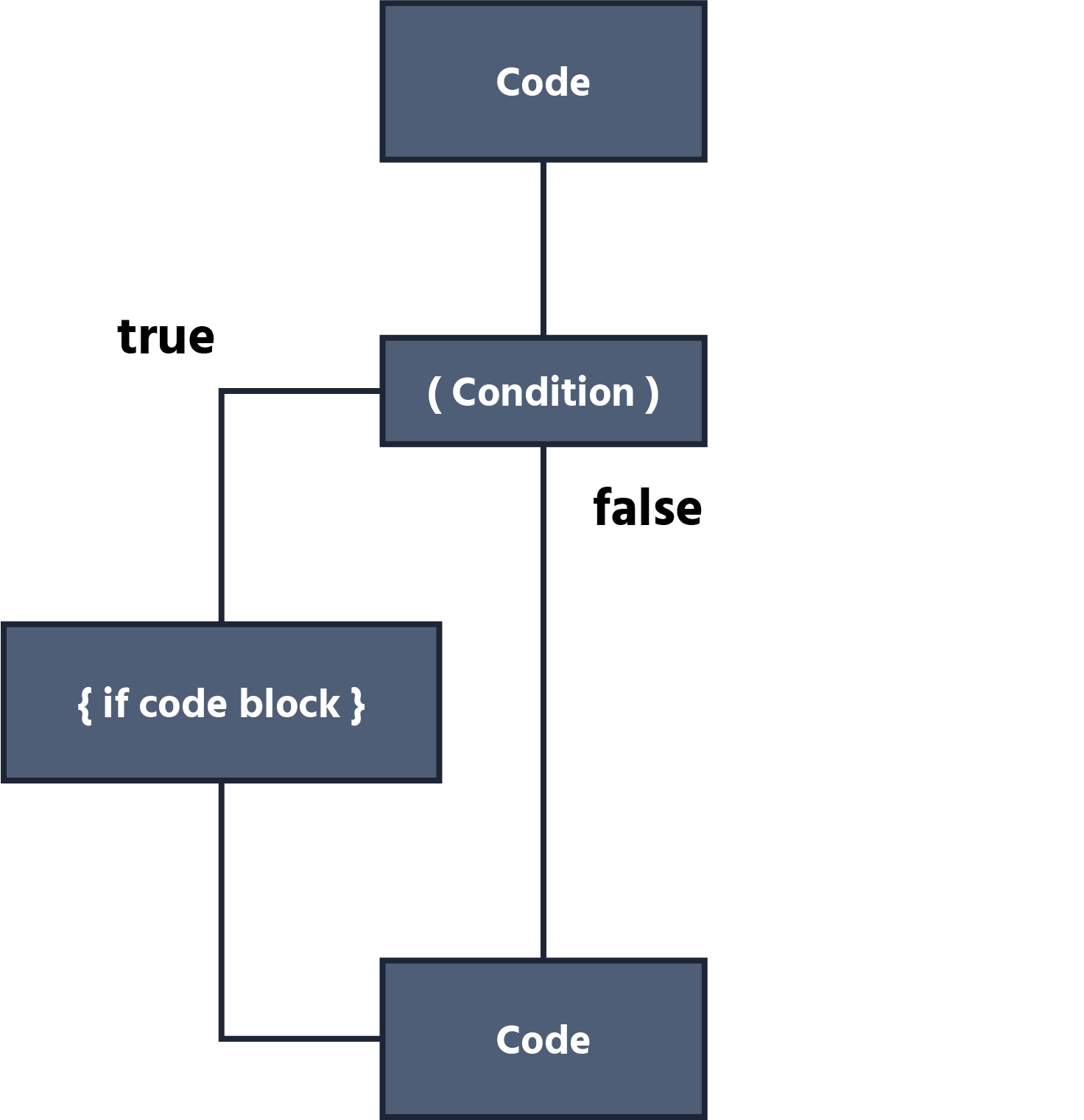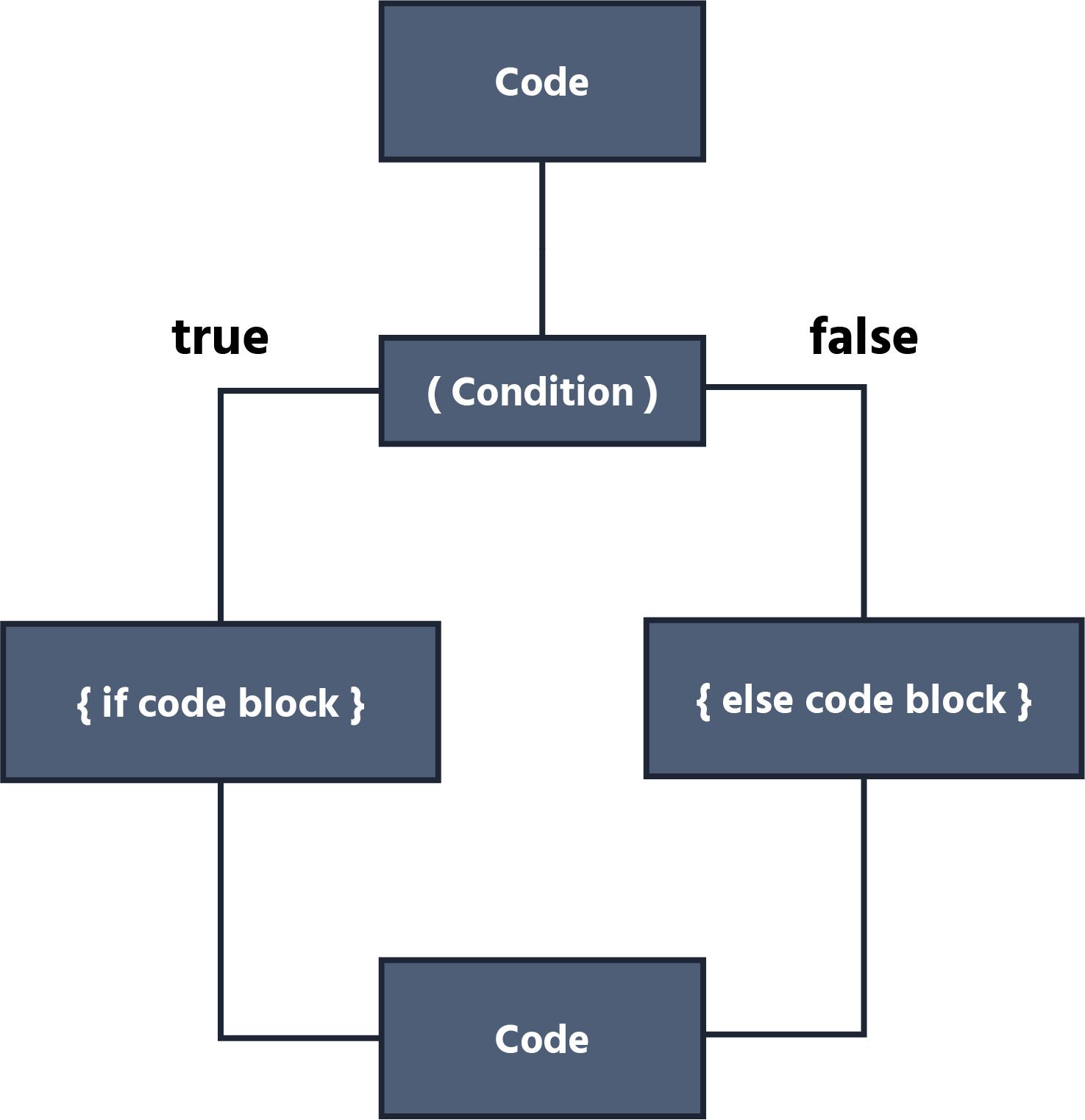Course Content

Java Basics

##If-Else Statement## Comparison Operators

To start, let's understand what comparison operators are.

The following are comparison operators: >, <, >=, <=, ==, !=. Let's quickly go through what each of them does:

• `>` (greater than) compares if the value on the left is greater than the value on the right.
• `<` (less than) compares if the value on the left is less than the value on the right.
• `>=` (greater than or equal to) compares if the value on the left is greater than or equal to the value on the right.
• `<=` (less than or equal to) compares if the value on the left is less than or equal to the value on the right.
• `==` (equal to) compares if the values on both sides are equal.
• `!=` (not equal to) compares if the values on both sides are not equal.

These operators are used to compare values and return a boolean result (`true` or `false`) based on the comparison.

## If Statement

The `if` statement is an essential part of any program. With the `if` statement, you can set conditions for your program. The syntax and the diagram of the `if` statement look like this:Main.javaLet's look at an example with real values:Main.javaIn this code, we establish a condition. If the value of `a` is greater than that of `b`, we display information about it. If the value of `b` exceeds `a`, we display different information about it.

But it doesn't look elegant when we have two separate if statements. We have a dedicated syntax for situations like this called the `if-else statement.` Let's see how we can improve the code above using the `if-else statement`:Main.javaWe can see how we improved our previous code using the `if-else statement.` In simple terms, we check if the value of variable `b` is greater, and if the returned value is false, we enter the else block, where we display a different message.

Here is the block scheme of If-Else Statement:Note

It's also worth noting that a boolean value should be stored inside the parentheses after the if-else statement. We can write it as boolean `bool = b > a;` In this case, the variable bool will hold the value `true`.

Let's examine a code fragment where we compare the values of two variables for equality:Main.javaHere, we are checking if the values of `a` and `b` are equal and displaying information about it. Since both `a` and `b` have a value of 10, the result will be `true`, so we display the corresponding message.

## else-if Statement

It is worth mentioning another statement called the `else-if` statement.

When we need to specify multiple different execution conditions, we can use the following syntax:Main.javaHere, we can see that we are checking two different conditions, and if none of them are satisfied, we enter the `else` block.

1. What is the result of this code?
2. What will be printed to console after code execution?

####What will be printed to console after code execution?Everything was clear?

Section 2. Chapter 5